Perimeter and MeasurementsPage 1

WATCH ALL SLIDES

Slide 1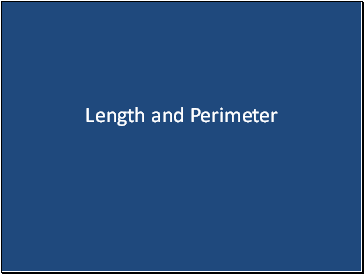Length and Perimeter

Slide 2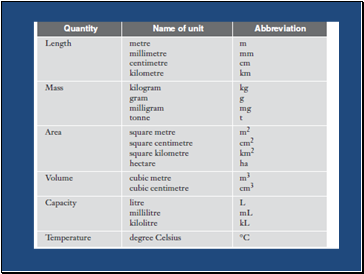Slide 3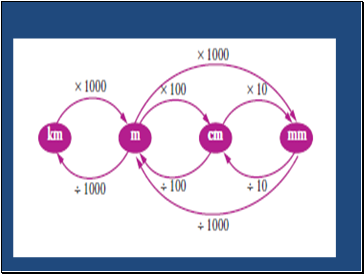Slide 4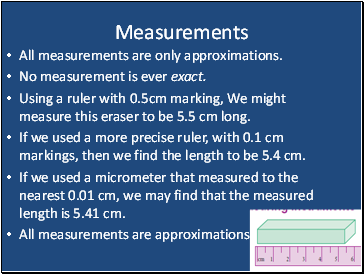Measurements

All measurements are only approximations.

No measurement is ever exact.

Using a ruler with 0.5cm marking, We might measure this eraser to be 5.5 cm long.

If we used a more precise ruler, with 0.1 cm markings, then we find the length to be 5.4 cm.

If we used a micrometer that measured to the nearest 0.01 cm, we may find that the measured length is 5.41 cm.

All measurements are approximations.

Slide 5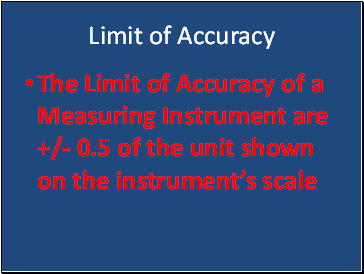Limit of Accuracy

The Limit of Accuracy of a Measuring Instrument are +/- 0.5 of the unit shown on the instrument’s scale

Slide 6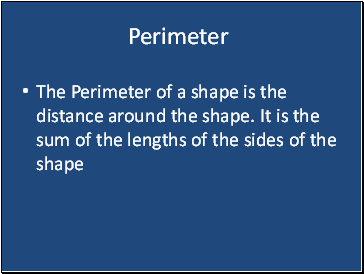Perimeter

The Perimeter of a shape is the distance around the shape. It is the sum of the lengths of the sides of the shape